+
Absolute Value Equations
Solving Linear Equations and Inequalities
0
of 0 possible points

# Absolute Value Equations

Author: Sophia Tutorial
##### Description:

Solve an absolute value equation.

(more)

Sophia’s self-paced online courses are a great way to save time and money as you earn credits eligible for transfer to many different colleges and universities.*

No credit card required

37 Sophia partners guarantee credit transfer.

299 Institutions have accepted or given pre-approval for credit transfer.

* The American Council on Education's College Credit Recommendation Service (ACE Credit®) has evaluated and recommended college credit for 33 of Sophia’s online courses. Many different colleges and universities consider ACE CREDIT recommendations in determining the applicability to their course and degree programs.

Tutorial
what's covered
1. Absolute Value Review
2. Equations in the form lxl
3. Equations in the form lmx + bl

# 1. Absolute Value Review

The absolute value of a number is the distance from zero on the number line. Distance is never a negative value, so absolute value never returns a negative.

We can look at the number line and see that the absolute value of a positive number and the absolute value of a negative number are both positive:Both –4 and 4 are 4 units away from zero on the number line. We can say that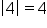and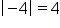.

This leads to a piecewise definition of absolute value:

formula
Absolute Value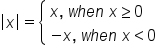This means that if x is zero or greater, the value of x does not change when we apply the absolute value. However, if x is negative, we change the sign of x when applying the absolute value, so as to make it positive.

# 2. Equations in the Form |x|

When we solve any absolute value equation, we just remember that the expression can be positive or negative, and still have the same absolute value. For this reason, we create two separate equations: one with a positive value, and one with a negative value, for the expression inside absolute value bars: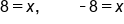Create two equations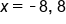Our solution

To solve absolute value equations, we can remove the absolute value bars only if we create two equations: one equation will look nearly identical to the original absolute value equation, while the second equation will consider the case when the expression is negative.

Next, we will consider more complex absolute value equations

# 3. Equations in the Form |mx + b|

Once again, we are going to solve this equation by creating two separate equations without absolute value bars. One equation will contain the expression exactly as it appears within the absolute value bars. The second equation must consider the case when the expression has the opposite value. That is, we reverse the sign on the other side of the equation: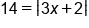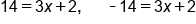Create two equations

Next, we solve each equation individually, and include both solutions for x as solutions to the absolute value equation.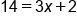First equation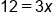Subtract 2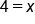Divide by 3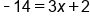Second equation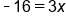Subtract 2Divide by 3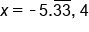Our Solution

big idea
Create two equations, each without absolute value bars. One equation will remain the same in every other respect, while the second equation will have the opposite value (reversed sign) on the other side of the equation.

summary
The absolute value of a number is its distance from 0 on the number line, and is always non-negative. We discussed two types of absolute value equations: equations in the form lxl and equations in the form lmx + bl. When solving absolute value equations, we must consider both the positive and negative values of the expression inside the absolute value bars, because they can equal the same value once we take the absolute value. Also, when solving absolute value equations, the first step is to isolate the absolute value expression on one side of the equation.

Formulas to Know
Absolute ValueRating